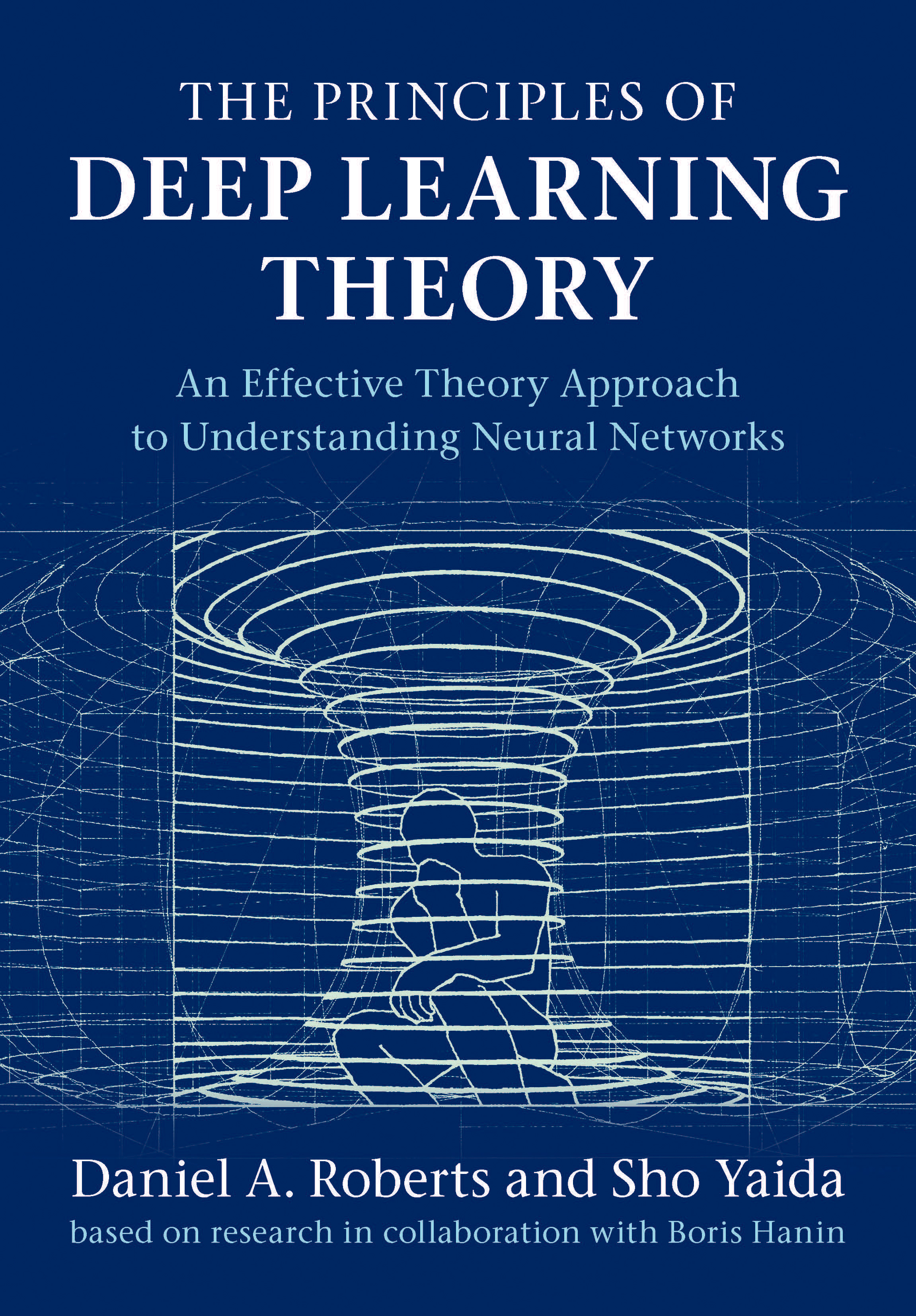Homepage: deeplearningtheory.com

# Lectures

An overview of the material in The Principles of Deep Learning Theory (PDLT) was given virtually in 2021 at the Princeton Deep Learning Theory (PDLT) Summer School by Dan Roberts and Sho Yaida.

### Lecture 1

TL;DW Dan gives an overview of the course and begins a discussion of training dynamics by covering linear models and kernel methods.

### Lecture 2

TL;DW Dan introduces the quadratic model as a minimal model of representation learning, and use gradient descent to solve the training dynamics. This extends kernel methods to “nearly-kernel methods.”

### Lecture 3

TL;DW Sho explains how to recursively compute the statistics of a deep and finite-width MLP at initialization. Due to the principle of sparsity, the distribution of the network output is tractable.

### Lecture 4

TL;DW Sho solves the layer-to-layer recursions derived before with the principle of criticality. We learn that the leading finite-width effects scale like the depth-to-width ratio of the network.

### Lecture 5

TL;DW By combining init statistics & training dynamics we get this. Then, Dan explain how MLPs *-polate, how to estimate a network’s optimal aspect ratio, and how to think about complexity.